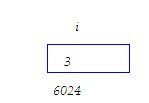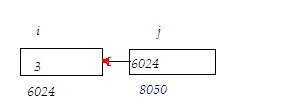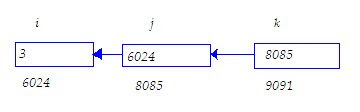## INDEX

### What is pointer ?

What is pointer ?
memory model

huge pointer

(1) What is pointer ?
Ans:
Pointer is variable which contain address of another variable.
e.g
1.
int i=3;
meaning:-2.
int i=3;
int *j;
j=&i;
meaning:Here j is pointer it contain 6024 which is address of another variable i.
3.
int i=3;
int *j;
int **k;
j=&i; //line 1
k=&j; //line 2

Meaning:Here both i and j are pointer where j contain 6024 which is address of variable I while j contain 8085 which is address of another variable which contain address of variable i.
Now

printf(“%d”, **k);
printf(“%d”,*j);
printf(“%d”,i);

Above all printf will give same output : 3
Explanation:* and & are canceled to each other i.e *&a=a
So **k
= **(&j) //from line 1
= *(*&j)
= *j //* and & are canceled to each other.
= *(&i) //from line 2
= *&i
= i
Thus **k = i ,hence **k is giving output 3

Same way *j = i
(2) What will output:void main()
{
int i=3;
int *j;
int **k;
j=&i;
k=&j;
printf(“%u %u %u”,i,j,k);
}
Ans:Here 6024 ,8085,9091 is any arbitrary address,it may be different.
Since content of i is 3,so first output will 3
Content of j is 6024 ,so second output will 6024
Content of k is 8085 ,so third output will 9091
Output: 3 6024 8085
Note:-Address is always a whole number ,which can not be negative so we generally is %u instead of %d
VISIT HERE

What is pointer ?

memory model

near pointer

far pointer